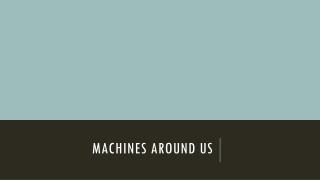DownloadDownload PresentationMachines around us

# Machines around us

Télécharger la présentation## Machines around us

- - - - - - - - - - - - - - - - - - - - - - - - - - - E N D - - - - - - - - - - - - - - - - - - - - - - - - - - -
##### Presentation Transcript

1. Machines around us

2. What can machines be used for? • Change the size of a force. • Make things speed up (Accelerate). • Change the direction of a force.

3. Think!! • Write three examples of machines you think are: • Force Multipliers • Speed Multipliers • Direction Changers

4. Levers • It is a simple machine that is made of a long rigid object (Timber or Metal rod) and a pivot (Fulcrum).

5. Types of Levers • First-class Lever • These levers have the fulcrum positioned between the effort and the load • Second-class Lever • These levers have the load positioned between the fulcrum and the effort • Third-class Lever • These levers have the effort positioned between the load and the fulcrum

7. First-class levers

8. Second-class levers

9. Third-class levers

10. Mechanical Advantage • The mechanical advantage of a machine tells you how much easier the task will be: • For when we are using levers this can also be calculated as: • If we had a mechanical advantage of 2 this means that: • - lift twice the load that you would normally be able to lift • - use half the force you would have otherwise needed to do a particular task

11. Example • Jess applies an effort of 150N to lift a rock of weight 600N using a long piece of wood as a lever. Calculate the mechanical advantage of the lever. • Load: 600N • Effort: 150N

12. Your turn • James lifts a 1000N object with a piece of wood, using an effort of 200N. Calculate the Mechanical Advantage.

13. Example • Con uses another stick to shift a second rock. The distance from his hand on the stick to the fulcrum is 120cm. The distance from the fulcrum to the rock is 40cm. Calculate the mechanical advantage.

14. Your turn Chloe lifts a box that is 100cm from the fulcrum. Her hand is placed 150cm from the fulcrum. Calculate the Mechanical advantage.

15. Questions  • Like always answer in your book in silence.

16. State the unit used to measure force Question 2

17. A lever is a simple machine that can increase the effort supplied to get a job done. Question 3. True or False.

18. If using a crowbar to lift a tree stump, then the tree stump is called the effort Question 4. True or False

19. A crowbar used as a lever rotates about a point called a fulcrum Question 5. True or False

20. When using a claw hammer to pull a nail out of a piece of wood, the hammer acts as a force multiplier Question 6. True or False

21. Calculate the size of the mechanical advantage for the systems above Question 7.

22. Inclined Planes

23. Ramps • If we lift an object directly it requires a large effort. • We can use a ramp or inclined plane to make it easier. • We use a smaller force over a longer distance